# Precalculus : Inverse Functions

## Example Questions

1 2 3 5 Next →

### Example Question #121 : Functions

Find the inverse function of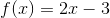.

Possible Answers: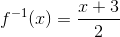None of the other answers.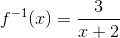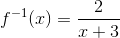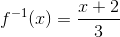Correct answer:Explanation:

To find the inverse you must reverse the variables and solve for y.

Reverse the variables: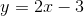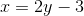Solve for y: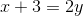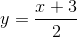### Example Question #122 : Functions

Are these two function inverses?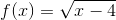and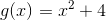.

Possible Answers:

G(x) does not have an inverse.

No

Cannot tell

Yes

F(x) does not have an inverse.

Correct answer:

Yes

Explanation:

One can ascertain if two functions have an inverse by finding the composition of both functions in turn. Each composition should equal x if the functions are indeed inverses of each other.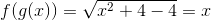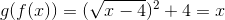The functions are inverses of each other.

### Example Question #123 : Functions

Find the inverse of the following equation: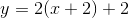Possible Answers: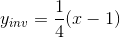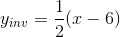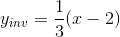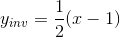Correct answer:Explanation:

To find the inverse of a function, replace the x any y positions:

Original Equation:Inversed Equation: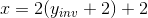Now solve for the inversed y value.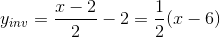### Example Question #124 : Functions

Find the inverse of the following equation: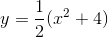Possible Answers: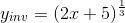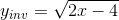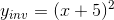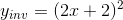Correct answer:Explanation:

To find the inverse of a function, replace the x any y positions:

Original Equation:Inversed Equation: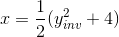Now solve for the inversed y value.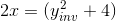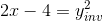### Example Question #125 : Functions

Find the inverse of the following equation: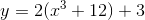Possible Answers: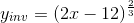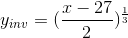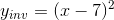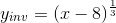Correct answer:Explanation:

To find the inverse of a function, replace the x any y positions:

Original Equation:Inversed Equation: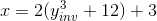Now solve for the inversed y value.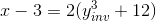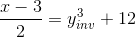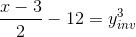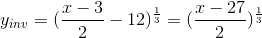### Example Question #126 : Functions

Determine the inverse function, given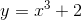Possible Answers: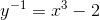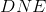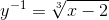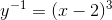Correct answer:Explanation:

In order to find the inverse function we

1. switch the variablesand2. solve for the newvariable

For the function...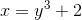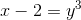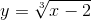Hence, the inverse function is1 2 3 5 Next →

### All Precalculus Resources